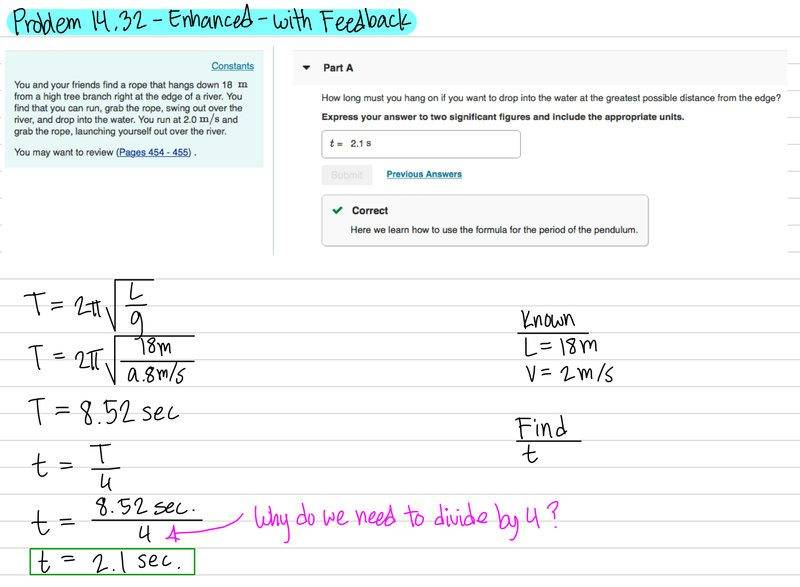# Period (T) of a pendulum

## Homework Statement

The question is in part 3 (image). Basically I used the formula for Period, T, of a pendulum of length L with free-fall acceleration g. I got 8.52, but I had to further divide this number by 4 to get the time the person must hang on in order to drop into the water at the greatest possible instance from the edge. Why does it need to be divided by 4? I know that leaving the answer to be equal to T is wrong because you'd be back to your original position. But why divide by 4?

Refer to part 3

## The Attempt at a Solution#### Attachments

Ssnow
Gold Member
Hi, ##T## is the time taken for a complete cycle (roundtrip), what you need is only the time from the equilibrium point to an extreme, so you must divide by four.

Ssnow

•asilvester635
Hi, ##T## is the time taken for a complete cycle (roundtrip), what you need is only the time from the equilibrium point to an extreme, so you must divide by four.

Ssnow
Thanks, it made so much sense when I drew a picture.